# `sklearn.linear_model`.LinearRegression¶

class `sklearn.linear_model.``LinearRegression`(fit_intercept=True, normalize=False, copy_X=True, n_jobs=1)[source]

Ordinary least squares Linear Regression.

Parameters: fit_intercept : boolean, optional, default True whether to calculate the intercept for this model. If set to False, no intercept will be used in calculations (e.g. data is expected to be already centered). normalize : boolean, optional, default False This parameter is ignored when `fit_intercept` is set to False. If True, the regressors X will be normalized before regression by subtracting the mean and dividing by the l2-norm. If you wish to standardize, please use `sklearn.preprocessing.StandardScaler` before calling `fit` on an estimator with `normalize=False`. copy_X : boolean, optional, default True If True, X will be copied; else, it may be overwritten. n_jobs : int, optional, default 1 The number of jobs to use for the computation. If -1 all CPUs are used. This will only provide speedup for n_targets > 1 and sufficient large problems. coef_ : array, shape (n_features, ) or (n_targets, n_features) Estimated coefficients for the linear regression problem. If multiple targets are passed during the fit (y 2D), this is a 2D array of shape (n_targets, n_features), while if only one target is passed, this is a 1D array of length n_features. intercept_ : array Independent term in the linear model.

Notes

From the implementation point of view, this is just plain Ordinary Least Squares (scipy.linalg.lstsq) wrapped as a predictor object.

Methods

 `fit`(X, y[, sample_weight]) Fit linear model. `get_params`([deep]) Get parameters for this estimator. `predict`(X) Predict using the linear model `score`(X, y[, sample_weight]) Returns the coefficient of determination R^2 of the prediction. `set_params`(**params) Set the parameters of this estimator.
`__init__`(fit_intercept=True, normalize=False, copy_X=True, n_jobs=1)[source]
`fit`(X, y, sample_weight=None)[source]

Fit linear model.

Parameters: X : numpy array or sparse matrix of shape [n_samples,n_features] Training data y : numpy array of shape [n_samples, n_targets] Target values. Will be cast to X’s dtype if necessary sample_weight : numpy array of shape [n_samples] Individual weights for each sample New in version 0.17: parameter sample_weight support to LinearRegression. self : returns an instance of self.
`get_params`(deep=True)[source]

Get parameters for this estimator.

Parameters: deep : boolean, optional If True, will return the parameters for this estimator and contained subobjects that are estimators. params : mapping of string to any Parameter names mapped to their values.
`predict`(X)[source]

Predict using the linear model

Parameters: X : {array-like, sparse matrix}, shape = (n_samples, n_features) Samples. C : array, shape = (n_samples,) Returns predicted values.
`score`(X, y, sample_weight=None)[source]

Returns the coefficient of determination R^2 of the prediction.

The coefficient R^2 is defined as (1 - u/v), where u is the residual sum of squares ((y_true - y_pred) ** 2).sum() and v is the total sum of squares ((y_true - y_true.mean()) ** 2).sum(). The best possible score is 1.0 and it can be negative (because the model can be arbitrarily worse). A constant model that always predicts the expected value of y, disregarding the input features, would get a R^2 score of 0.0.

Parameters: X : array-like, shape = (n_samples, n_features) Test samples. y : array-like, shape = (n_samples) or (n_samples, n_outputs) True values for X. sample_weight : array-like, shape = [n_samples], optional Sample weights. score : float R^2 of self.predict(X) wrt. y.
`set_params`(**params)[source]

Set the parameters of this estimator.

The method works on simple estimators as well as on nested objects (such as pipelines). The latter have parameters of the form `<component>__<parameter>` so that it’s possible to update each component of a nested object.

Returns: self :

## Examples using `sklearn.linear_model.LinearRegression`¶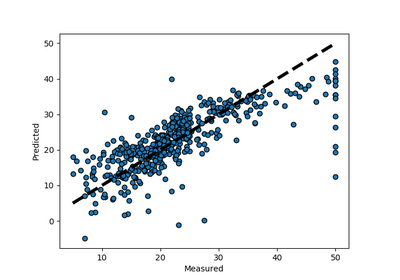Plotting Cross-Validated Predictions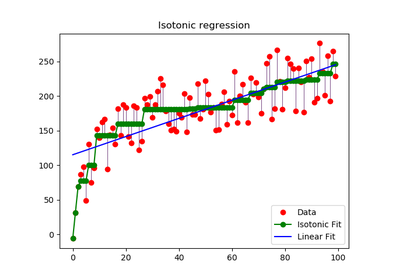Isotonic Regression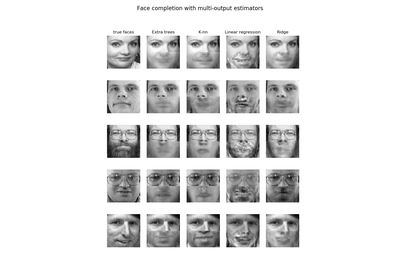Face completion with a multi-output estimators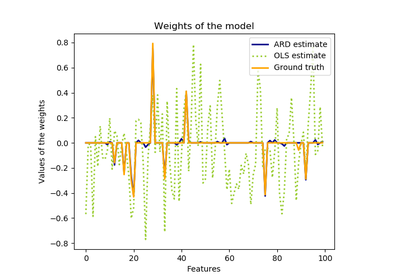Automatic Relevance Determination Regression (ARD)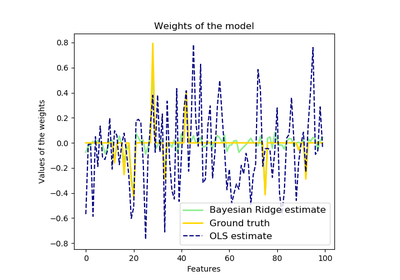Bayesian Ridge Regression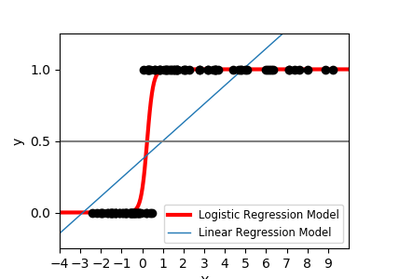Logistic function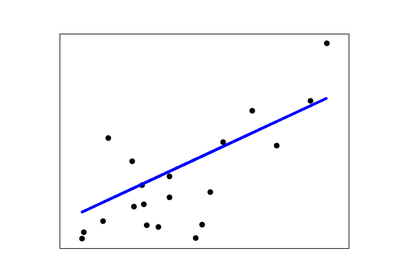Linear Regression Example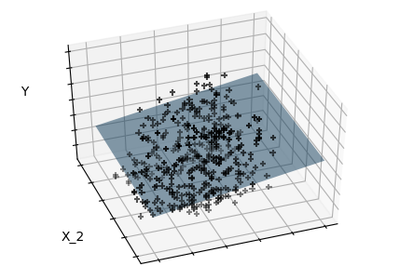Sparsity Example: Fitting only features 1 and 2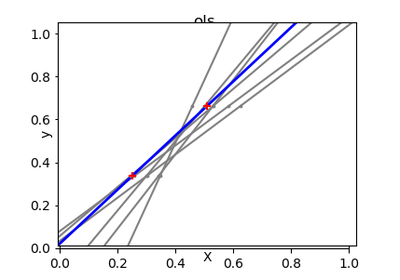Ordinary Least Squares and Ridge Regression Variance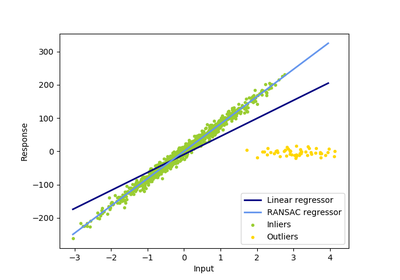Robust linear model estimation using RANSAC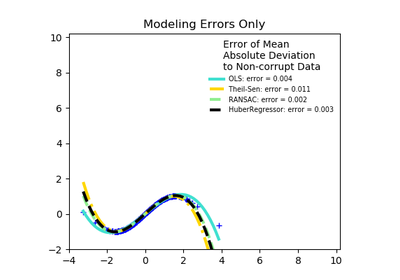Robust linear estimator fitting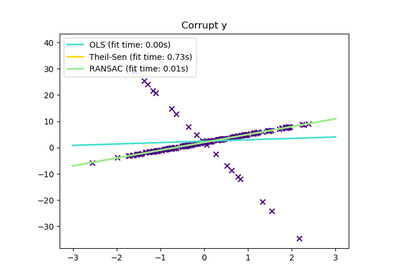Theil-Sen Regression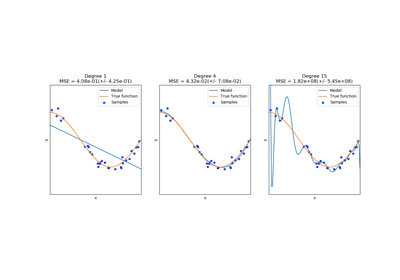Underfitting vs. Overfitting